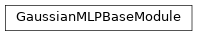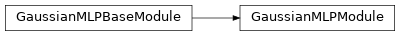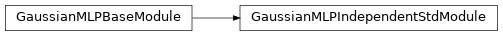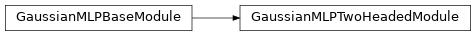# `garage.torch.modules.gaussian_mlp_module`¶

GaussianMLPModule.

class GaussianMLPBaseModule(input_dim, output_dim, hidden_sizes=(32, 32), *, hidden_nonlinearity=torch.tanh, hidden_w_init=nn.init.xavier_uniform_, hidden_b_init=nn.init.zeros_, output_nonlinearity=None, output_w_init=nn.init.xavier_uniform_, output_b_init=nn.init.zeros_, learn_std=True, init_std=1.0, min_std=1e-06, max_std=None, std_hidden_sizes=(32, 32), std_hidden_nonlinearity=torch.tanh, std_hidden_w_init=nn.init.xavier_uniform_, std_hidden_b_init=nn.init.zeros_, std_output_nonlinearity=None, std_output_w_init=nn.init.xavier_uniform_, std_parameterization='exp', layer_normalization=False, normal_distribution_cls=Normal)

Bases: `torch.nn.Module`Base of GaussianMLPModel.

Parameters
• input_dim (int) – Input dimension of the model.

• output_dim (int) – Output dimension of the model.

• hidden_sizes (list[int]) – Output dimension of dense layer(s) for the MLP for mean. For example, (32, 32) means the MLP consists of two hidden layers, each with 32 hidden units.

• hidden_nonlinearity (callable) – Activation function for intermediate dense layer(s). It should return a torch.Tensor. Set it to None to maintain a linear activation.

• hidden_w_init (callable) – Initializer function for the weight of intermediate dense layer(s). The function should return a torch.Tensor.

• hidden_b_init (callable) – Initializer function for the bias of intermediate dense layer(s). The function should return a torch.Tensor.

• output_nonlinearity (callable) – Activation function for output dense layer. It should return a torch.Tensor. Set it to None to maintain a linear activation.

• output_w_init (callable) – Initializer function for the weight of output dense layer(s). The function should return a torch.Tensor.

• output_b_init (callable) – Initializer function for the bias of output dense layer(s). The function should return a torch.Tensor.

• learn_std (bool) – Is std trainable.

• init_std (float) – Initial value for std. (plain value - not log or exponentiated).

• std_hidden_sizes (list[int]) – Output dimension of dense layer(s) for the MLP for std. For example, (32, 32) means the MLP consists of two hidden layers, each with 32 hidden units.

• min_std (float) – If not None, the std is at least the value of min_std, to avoid numerical issues (plain value - not log or exponentiated).

• max_std (float) – If not None, the std is at most the value of max_std, to avoid numerical issues (plain value - not log or exponentiated).

• std_hidden_nonlinearity (callable) – Nonlinearity for each hidden layer in the std network.

• std_hidden_w_init (callable) – Initializer function for the weight of hidden layer (s).

• std_hidden_b_init (callable) – Initializer function for the bias of intermediate dense layer(s).

• std_output_nonlinearity (callable) – Activation function for output dense layer in the std network. It should return a torch.Tensor. Set it to None to maintain a linear activation.

• std_output_w_init (callable) – Initializer function for the weight of output dense layer(s) in the std network.

• std_parameterization (str) –

How the std should be parametrized. There are two options: - exp: the logarithm of the std will be stored, and applied a

exponential transformation.

• softplus: the std will be computed as log(1+exp(x)).

• layer_normalization (bool) – Bool for using layer normalization or not.

• normal_distribution_cls (torch.distribution) – normal distribution class to be constructed and returned by a call to forward. By default, is torch.distributions.Normal.

to(*args, **kwargs)

Move the module to the specified device.

Parameters
• *args – args to pytorch to function.

• **kwargs – keyword args to pytorch to function.

forward(*inputs)

Forward method.

Parameters

*inputs – Input to the module.

Returns

Independent

distribution.

Return type

torch.distributions.independent.Independent

class GaussianMLPModule(input_dim, output_dim, hidden_sizes=(32, 32), *, hidden_nonlinearity=torch.tanh, hidden_w_init=nn.init.xavier_uniform_, hidden_b_init=nn.init.zeros_, output_nonlinearity=None, output_w_init=nn.init.xavier_uniform_, output_b_init=nn.init.zeros_, learn_std=True, init_std=1.0, min_std=1e-06, max_std=None, std_parameterization='exp', layer_normalization=False, normal_distribution_cls=Normal)GaussianMLPModule that mean and std share the same network.

Parameters
• input_dim (int) – Input dimension of the model.

• output_dim (int) – Output dimension of the model.

• hidden_sizes (list[int]) – Output dimension of dense layer(s) for the MLP for mean. For example, (32, 32) means the MLP consists of two hidden layers, each with 32 hidden units.

• hidden_nonlinearity (callable) – Activation function for intermediate dense layer(s). It should return a torch.Tensor. Set it to None to maintain a linear activation.

• hidden_w_init (callable) – Initializer function for the weight of intermediate dense layer(s). The function should return a torch.Tensor.

• hidden_b_init (callable) – Initializer function for the bias of intermediate dense layer(s). The function should return a torch.Tensor.

• output_nonlinearity (callable) – Activation function for output dense layer. It should return a torch.Tensor. Set it to None to maintain a linear activation.

• output_w_init (callable) – Initializer function for the weight of output dense layer(s). The function should return a torch.Tensor.

• output_b_init (callable) – Initializer function for the bias of output dense layer(s). The function should return a torch.Tensor.

• learn_std (bool) – Is std trainable.

• init_std (float) – Initial value for std. (plain value - not log or exponentiated).

• min_std (float) – If not None, the std is at least the value of min_std, to avoid numerical issues (plain value - not log or exponentiated).

• max_std (float) – If not None, the std is at most the value of max_std, to avoid numerical issues (plain value - not log or exponentiated).

• std_parameterization (str) –

How the std should be parametrized. There are two options: - exp: the logarithm of the std will be stored, and applied a

exponential transformation

• softplus: the std will be computed as log(1+exp(x))

• layer_normalization (bool) – Bool for using layer normalization or not.

• normal_distribution_cls (torch.distribution) – normal distribution class to be constructed and returned by a call to forward. By default, is torch.distributions.Normal.

to(*args, **kwargs)

Move the module to the specified device.

Parameters
• *args – args to pytorch to function.

• **kwargs – keyword args to pytorch to function.

forward(*inputs)

Forward method.

Parameters

*inputs – Input to the module.

Returns

Independent

distribution.

Return type

torch.distributions.independent.Independent

class GaussianMLPIndependentStdModule(input_dim, output_dim, hidden_sizes=(32, 32), *, hidden_nonlinearity=torch.tanh, hidden_w_init=nn.init.xavier_uniform_, hidden_b_init=nn.init.zeros_, output_nonlinearity=None, output_w_init=nn.init.xavier_uniform_, output_b_init=nn.init.zeros_, learn_std=True, init_std=1.0, min_std=1e-06, max_std=None, std_hidden_sizes=(32, 32), std_hidden_nonlinearity=torch.tanh, std_hidden_w_init=nn.init.xavier_uniform_, std_hidden_b_init=nn.init.zeros_, std_output_nonlinearity=None, std_output_w_init=nn.init.xavier_uniform_, std_parameterization='exp', layer_normalization=False, normal_distribution_cls=Normal)GaussianMLPModule which has two different mean and std network.

Parameters
• input_dim (int) – Input dimension of the model.

• output_dim (int) – Output dimension of the model.

• hidden_sizes (list[int]) – Output dimension of dense layer(s) for the MLP for mean. For example, (32, 32) means the MLP consists of two hidden layers, each with 32 hidden units.

• hidden_nonlinearity (callable) – Activation function for intermediate dense layer(s). It should return a torch.Tensor. Set it to None to maintain a linear activation.

• hidden_w_init (callable) – Initializer function for the weight of intermediate dense layer(s). The function should return a torch.Tensor.

• hidden_b_init (callable) – Initializer function for the bias of intermediate dense layer(s). The function should return a torch.Tensor.

• output_nonlinearity (callable) – Activation function for output dense layer. It should return a torch.Tensor. Set it to None to maintain a linear activation.

• output_w_init (callable) – Initializer function for the weight of output dense layer(s). The function should return a torch.Tensor.

• output_b_init (callable) – Initializer function for the bias of output dense layer(s). The function should return a torch.Tensor.

• learn_std (bool) – Is std trainable.

• init_std (float) – Initial value for std. (plain value - not log or exponentiated).

• min_std (float) – If not None, the std is at least the value of min_std, to avoid numerical issues (plain value - not log or exponentiated).

• max_std (float) – If not None, the std is at most the value of max_std, to avoid numerical issues (plain value - not log or exponentiated).

• std_hidden_sizes (list[int]) – Output dimension of dense layer(s) for the MLP for std. For example, (32, 32) means the MLP consists of two hidden layers, each with 32 hidden units.

• std_hidden_nonlinearity (callable) – Nonlinearity for each hidden layer in the std network.

• std_hidden_w_init (callable) – Initializer function for the weight of hidden layer (s).

• std_hidden_b_init (callable) – Initializer function for the bias of intermediate dense layer(s).

• std_output_nonlinearity (callable) – Activation function for output dense layer in the std network. It should return a torch.Tensor. Set it to None to maintain a linear activation.

• std_output_w_init (callable) – Initializer function for the weight of output dense layer(s) in the std network.

• std_parameterization (str) –

How the std should be parametrized. There are two options: - exp: the logarithm of the std will be stored, and applied a

exponential transformation

• softplus: the std will be computed as log(1+exp(x))

• layer_normalization (bool) – Bool for using layer normalization or not.

• normal_distribution_cls (torch.distribution) – normal distribution class to be constructed and returned by a call to forward. By default, is torch.distributions.Normal.

to(*args, **kwargs)

Move the module to the specified device.

Parameters
• *args – args to pytorch to function.

• **kwargs – keyword args to pytorch to function.

forward(*inputs)

Forward method.

Parameters

*inputs – Input to the module.

Returns

Independent

distribution.

Return type

torch.distributions.independent.Independent

class GaussianMLPTwoHeadedModule(input_dim, output_dim, hidden_sizes=(32, 32), *, hidden_nonlinearity=torch.tanh, hidden_w_init=nn.init.xavier_uniform_, hidden_b_init=nn.init.zeros_, output_nonlinearity=None, output_w_init=nn.init.xavier_uniform_, output_b_init=nn.init.zeros_, learn_std=True, init_std=1.0, min_std=1e-06, max_std=None, std_parameterization='exp', layer_normalization=False, normal_distribution_cls=Normal)GaussianMLPModule which has only one mean network.

Parameters
• input_dim (int) – Input dimension of the model.

• output_dim (int) – Output dimension of the model.

• hidden_sizes (list[int]) – Output dimension of dense layer(s) for the MLP for mean. For example, (32, 32) means the MLP consists of two hidden layers, each with 32 hidden units.

• hidden_nonlinearity (callable) – Activation function for intermediate dense layer(s). It should return a torch.Tensor. Set it to None to maintain a linear activation.

• hidden_w_init (callable) – Initializer function for the weight of intermediate dense layer(s). The function should return a torch.Tensor.

• hidden_b_init (callable) – Initializer function for the bias of intermediate dense layer(s). The function should return a torch.Tensor.

• output_nonlinearity (callable) – Activation function for output dense layer. It should return a torch.Tensor. Set it to None to maintain a linear activation.

• output_w_init (callable) – Initializer function for the weight of output dense layer(s). The function should return a torch.Tensor.

• output_b_init (callable) – Initializer function for the bias of output dense layer(s). The function should return a torch.Tensor.

• learn_std (bool) – Is std trainable.

• init_std (float) – Initial value for std. (plain value - not log or exponentiated).

• min_std (float) – If not None, the std is at least the value of min_std, to avoid numerical issues (plain value - not log or exponentiated).

• max_std (float) – If not None, the std is at most the value of max_std, to avoid numerical issues (plain value - not log or exponentiated).

• std_parameterization (str) –

How the std should be parametrized. There are two options: - exp: the logarithm of the std will be stored, and applied a

exponential transformation

• softplus: the std will be computed as log(1+exp(x))

• layer_normalization (bool) – Bool for using layer normalization or not.

• normal_distribution_cls (torch.distribution) – normal distribution class to be constructed and returned by a call to forward. By default, is torch.distributions.Normal.

to(*args, **kwargs)

Move the module to the specified device.

Parameters
• *args – args to pytorch to function.

• **kwargs – keyword args to pytorch to function.

forward(*inputs)

Forward method.

Parameters

*inputs – Input to the module.

Returns

Independent

distribution.

Return type

torch.distributions.independent.Independent